# Money never sleeps, pal.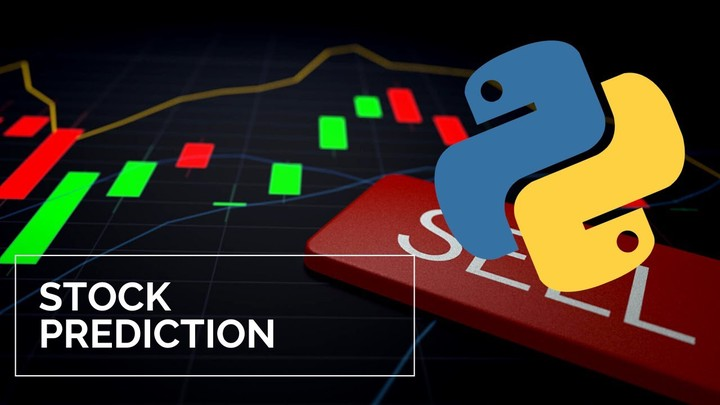# PROJECT DETAILS

This project is on stock trading, specifically the SP500 & some very in demand stocks such as Apple, Amazon, and Google. The script will show the path that I took in order to get the most from the dataset visually and then explore a model that I found that could predict Apple’s stock to 92% accuracy. I then used the same model to the SP500 to show the ability to an index by using an LSTM model in keras. I hope you enjoy it and check out the code at my Github with the button above or share it with your network on Linkedin!

# Math (Skip if you only want to see the fun stuff)

Ridge Regression is a way to create a model, when the number of predicator variables exceed the number of observations, or when the dataset has multicollinearity 🔔 (correlations between predictor variables). Also, the Ridge Regression is a L2 regression which add a penalty. The penalty is equal to the squares of the magnitude of coefficients. Penalty = Losing Money

So it is a perfect fit!

LSTM & Time Series LSTM is a recurrent neural network (RNN) that is trained by using Backpropagation through time and overcomes the vanishing gradient problem.

Long-Strong-Term Memory (LSTM) is the next generation of Recurrent Neural Network (RNN) used in deep learning for its optimized architecture to easily capture the pattern in sequential data aka STOCKS

# 🍻

Cheers! Now lets begin!!

# IMPORT DATASETS AND LIBRARIES

``````# Data Maniupulation
import pandas as pd
import matplotlib.pyplot as plt
import numpy as np

# Data Visualization
import plotly.figure_factory as ff
import plotly.express as px

# Modeling
from sklearn.preprocessing import MinMaxScaler
from sklearn.linear_model import LinearRegression
from sklearn.model_selection import train_test_split
from sklearn.linear_model import Ridge
from tensorflow import keras

from copy import copy
from scipy import stats
``````
``````# Stock prices data

# Stocks volume data
``````
``````# Sort the data based on Date
stocks_df = stocks_df.sort_values('Date')
``````
``````# Sort the volume data based on Date
stocks_vol_df = stocks_vol_df.sort_values('Date')
``````

# PERFORM EXPLORATORY DATA ANALYSIS AND VISUALIZATION

``````# Function to normalize stock prices based on their initial price
def normalize(df):
x = df.copy()
for i in x.columns[1:]:
x[i] = x[i]/x[i]
return x
``````
``````# Function to plot interactive plots using Plotly Express
def interactive_plot(df, title):
fig = px.line(title = title)
for i in df.columns[1:]:
fig.add_scatter(x = df['Date'], y = df[i], name =i)
fig.show()
``````
``````# plot interactive chart for stocks data
#interactive_plot(stocks_df, 'Stock Prices')
``````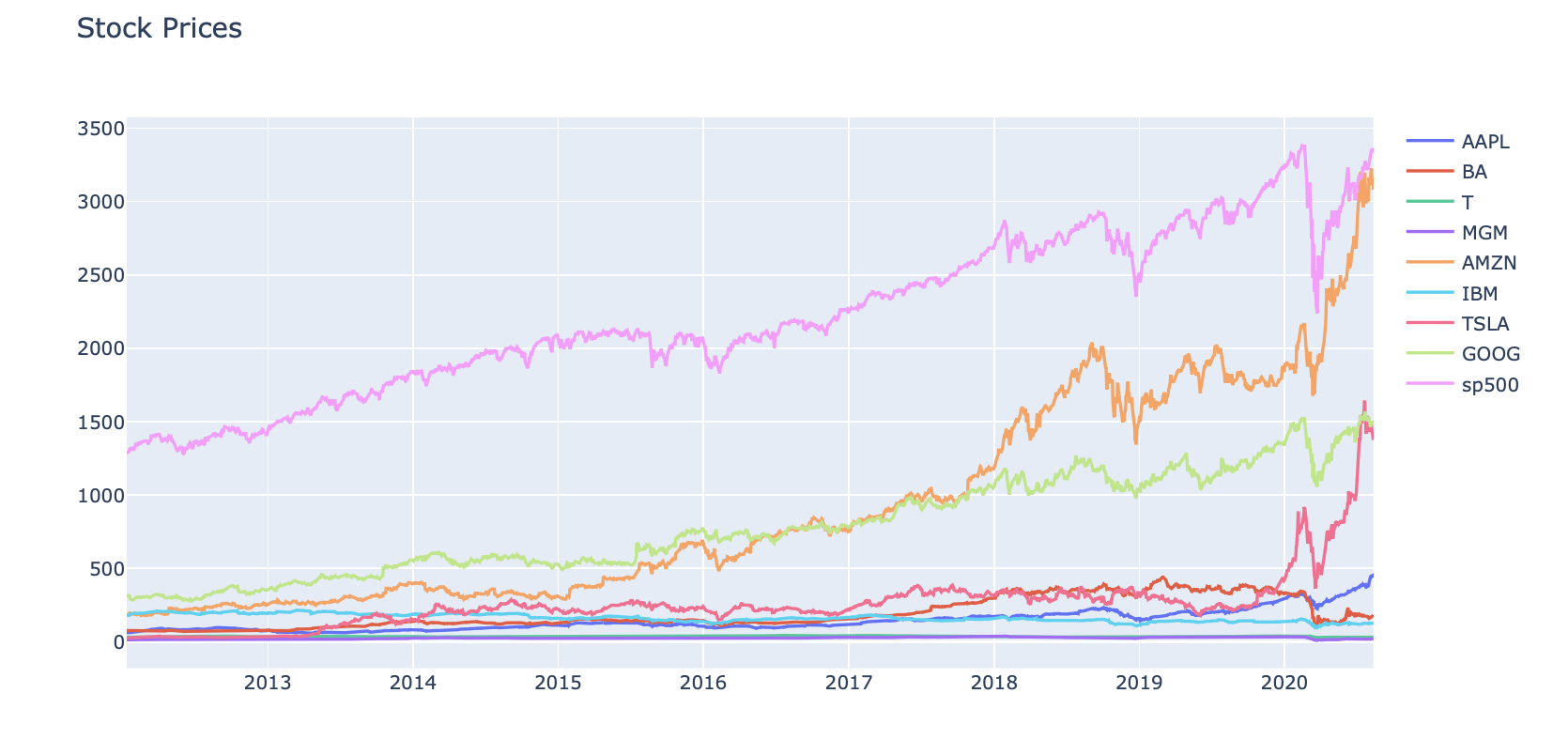``````#interactive_plot(normalize(stocks_df), 'Normalize Stock Prices')
``````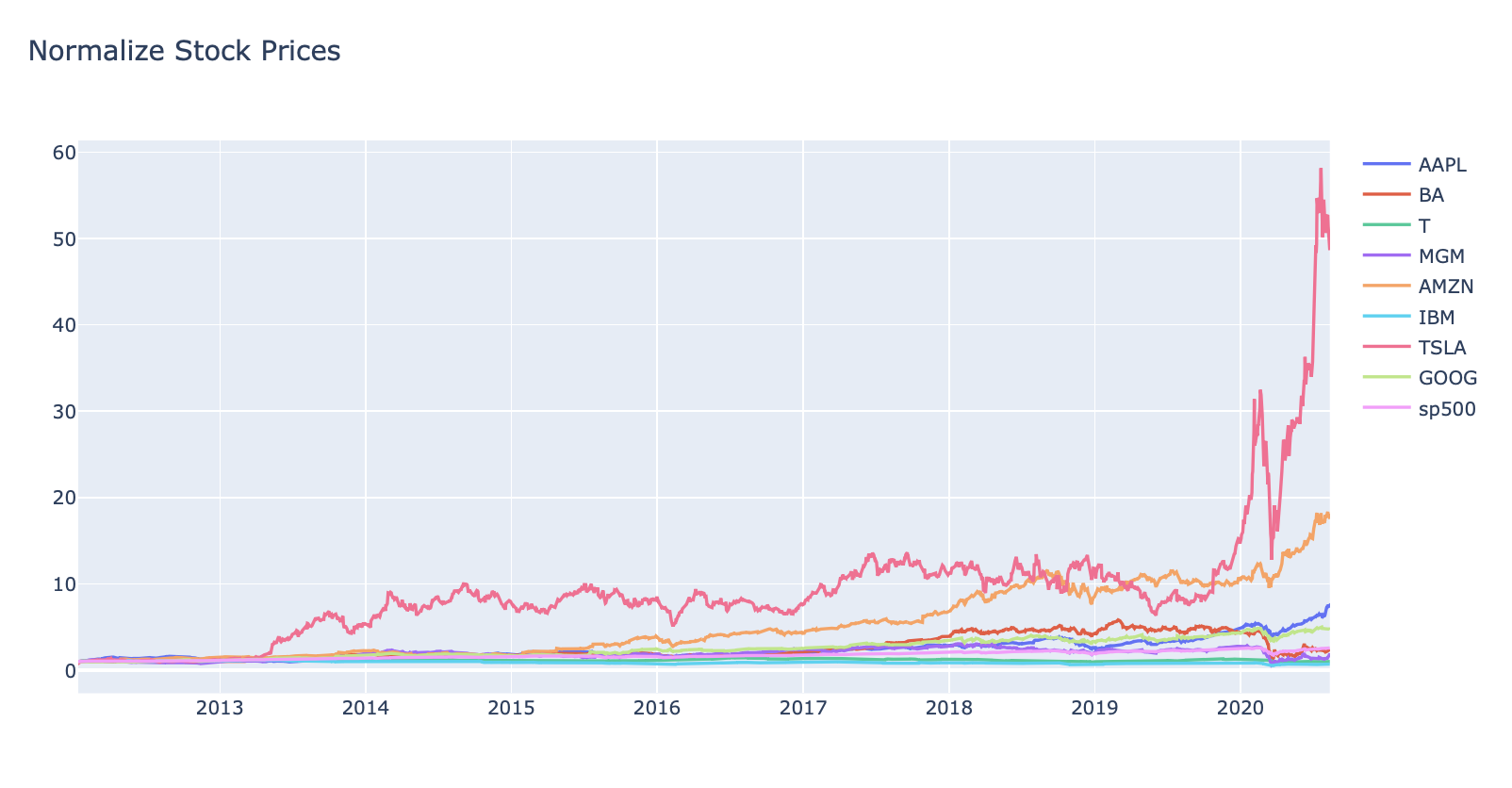``````#interactive_plot(stocks_vol_df, 'Stocks Volume')
``````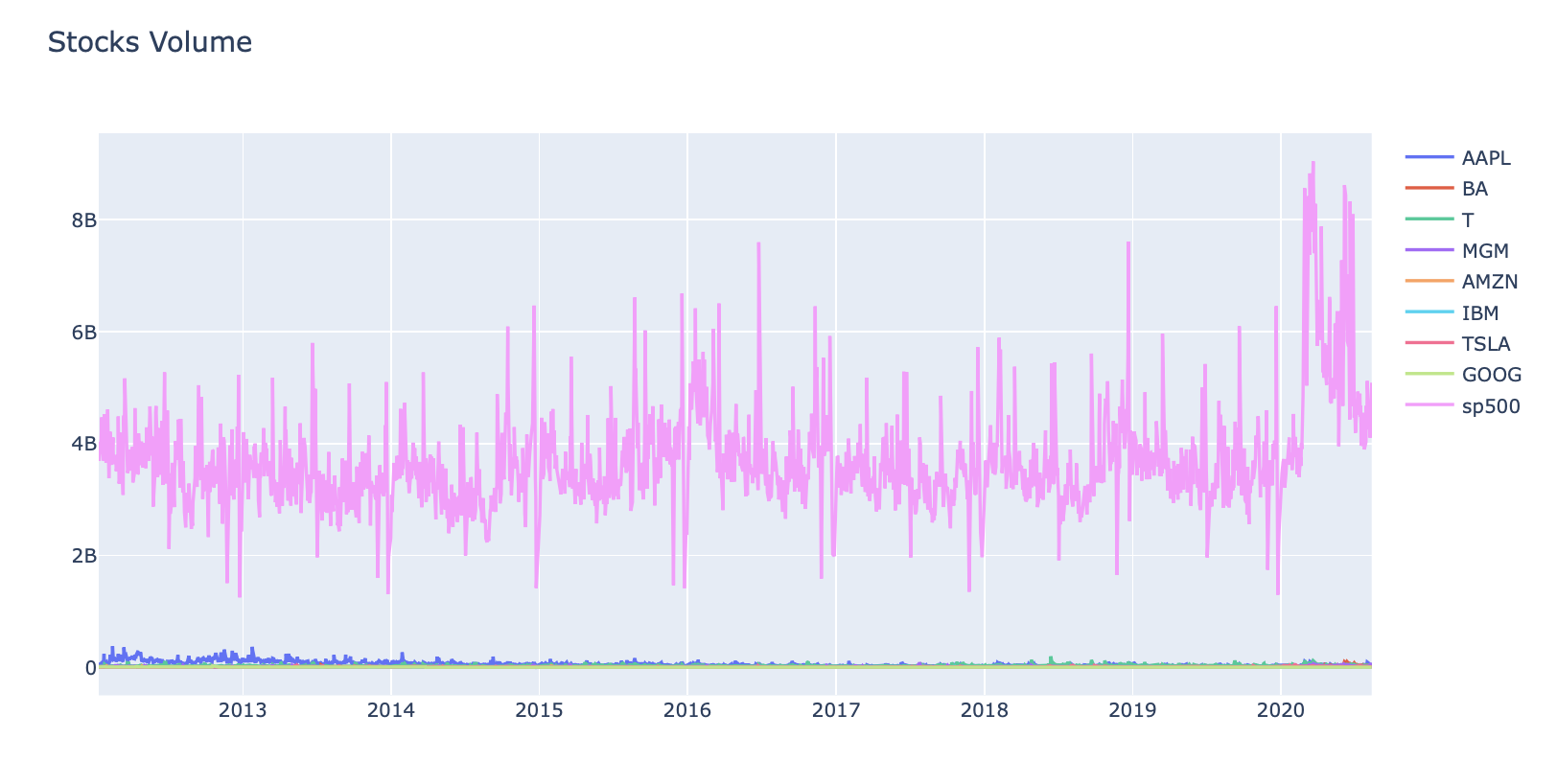``````#interactive_plot(normalize(stocks_vol_df), 'Normalizes Stock Volume')
``````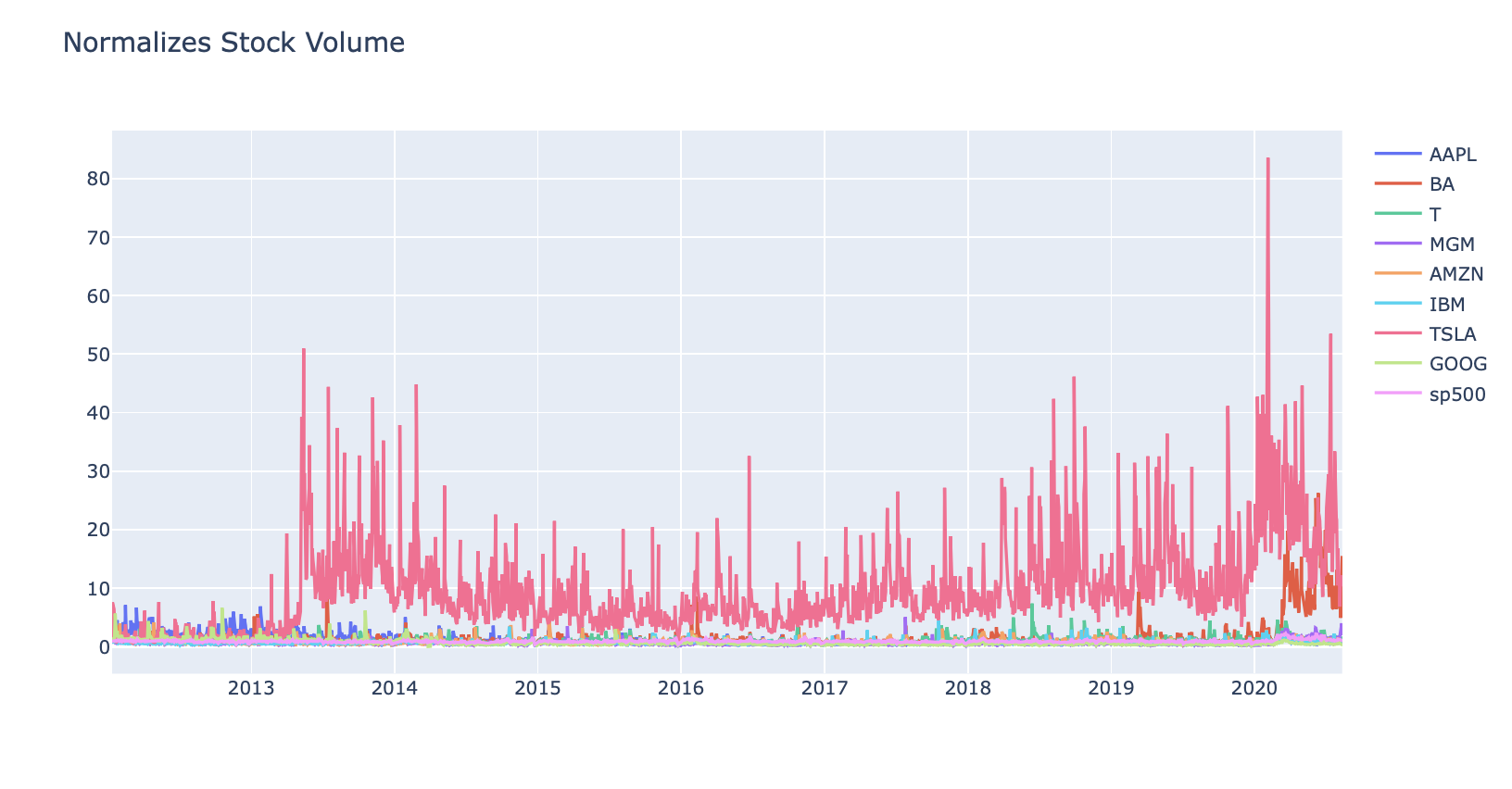# PREPARE THE DATA BEFORE TRAINING THE MODEL

``````# Function to concatenate the date, stock price, and volume in one dataframe
def individual_stock(price_df, vol_df, name):
return pd.DataFrame({'Date': price_df['Date'], 'Close': price_df[name], 'Volume': vol_df[name]})
``````
``````# Function to return the input/output (target) data for model
# Note that our goal is to predict the future stock price
# Target stock price today will be tomorrow's price
n = 1
data['Target'] = data[['Close']].shift(-n)
return data
``````

#### If you want to view SP 500 / AMZN / ETC: Change ‘APPL’ HERE!

``````# Let's test the functions and get individual stock prices and volumes for AAPL
price_volume_df = individual_stock(stocks_df, stocks_vol_df, 'AAPL')
price_volume_df
``````

Date Close Volume
0 2012-01-12 60.198570 53146800
1 2012-01-13 59.972858 56505400
2 2012-01-17 60.671429 60724300
3 2012-01-18 61.301430 69197800
4 2012-01-19 61.107143 65434600
... ... ... ...
2154 2020-08-05 440.250000 30498000
2155 2020-08-06 455.609985 50607200
2156 2020-08-07 444.450012 49453300
2157 2020-08-10 450.910004 53100900
2158 2020-08-11 437.500000 46871100

2159 rows × 3 columns

``````price_volume_target_df = trading_window(price_volume_df)
price_volume_target_df
``````

Date Close Volume Target
0 2012-01-12 60.198570 53146800 59.972858
1 2012-01-13 59.972858 56505400 60.671429
2 2012-01-17 60.671429 60724300 61.301430
3 2012-01-18 61.301430 69197800 61.107143
4 2012-01-19 61.107143 65434600 60.042858
... ... ... ... ...
2154 2020-08-05 440.250000 30498000 455.609985
2155 2020-08-06 455.609985 50607200 444.450012
2156 2020-08-07 444.450012 49453300 450.910004
2157 2020-08-10 450.910004 53100900 437.500000
2158 2020-08-11 437.500000 46871100 NaN

2159 rows × 4 columns

``````# Remove the last row as it will be a null value
price_volume_target_df = price_volume_target_df[:-1]
price_volume_target_df
``````

Date Close Volume Target
0 2012-01-12 60.198570 53146800 59.972858
1 2012-01-13 59.972858 56505400 60.671429
2 2012-01-17 60.671429 60724300 61.301430
3 2012-01-18 61.301430 69197800 61.107143
4 2012-01-19 61.107143 65434600 60.042858
... ... ... ... ...
2153 2020-08-04 438.660004 43267900 440.250000
2154 2020-08-05 440.250000 30498000 455.609985
2155 2020-08-06 455.609985 50607200 444.450012
2156 2020-08-07 444.450012 49453300 450.910004
2157 2020-08-10 450.910004 53100900 437.500000

2158 rows × 4 columns

``````# Scale the data
sc = MinMaxScaler(feature_range = (0,1))
price_volume_target_scaled_df = sc.fit_transform(price_volume_target_df.drop(columns = ['Date']))
``````
``````# Create Feature and Target
X = price_volume_target_scaled_df[:, :2]
y = price_volume_target_scaled_df[:, 2:]
``````
``````price_volume_target_scaled_df.shape
``````
``````(2158, 3)
``````
``````X.shape, y.shape
``````
``````((2158, 2), (2158, 1))
``````

### Note that we did not use train test split with it’s default settings since it shuffles the data

``````split = int(0.75 * len(X))
X_train = X[:split]
y_train = y[:split]
X_test = X[split:]
y_test = y[split:]
``````
``````X_train.shape, y_train.shape
``````
``````((1618, 2), (1618, 1))
``````
``````X_test.shape, y_test.shape
``````
``````((540, 2), (540, 1))
``````
``````# Define a data plotting function
print('''
APPLE
''')

def show_plot(data, title):
plt.figure(figsize = (13, 5))
plt.plot(data, linewidth = 3)
plt.title(title)
plt.xlabel(xlabel= 'Data Variable')
plt.ylabel(ylabel= 'Accuracy Relativity to 1' )
plt.grid()

show_plot(X_train, 'Training Data')
show_plot(X_test, 'Testing Data')
``````
``````                                            APPLE
``````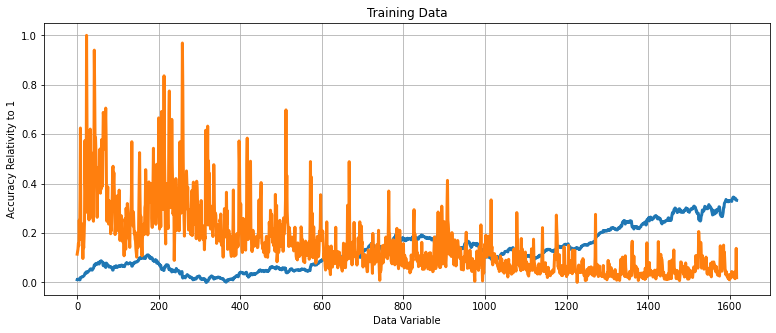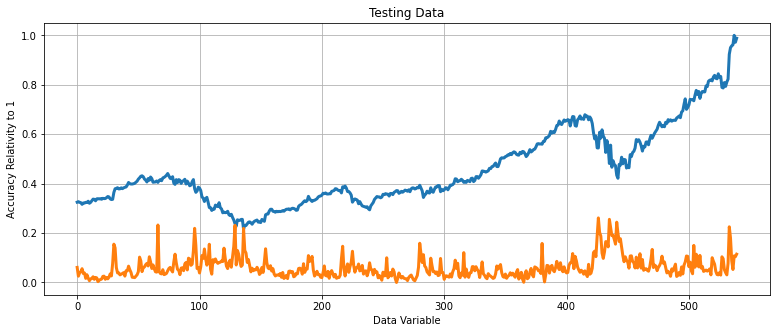# BUILD AND TRAIN A RIDGE LINEAR REGRESSION MODEL

``````regression_model = Ridge()

# Test the model and calculate its accuracy
regression_model.fit(X_train, y_train)

# Make Prediction
lr_accuracy = regression_model.score(X_test, y_test)
print('Ridge Regression Score:', lr_accuracy)
``````
``````Ridge Regression Score: 0.9311227075637692
``````
``````# Append the predicted values into a list
predicted_prices = regression_model.predict(X)
``````
``````predicted = []
for i in predicted_prices:
predicted.append(i)
``````
``````# Append the close values to the list
close = []
for i in price_volume_target_scaled_df:
close.append(i)
``````
``````# Create a dataframe based on the dates in the individual stock data
df_predicted = price_volume_target_df[['Date']]
``````
``````# Add the close values to the dataframe
df_predicted['Close'] = close
``````
``````# Add the predicted values to the dataframe
df_predicted['Prediction'] = predicted
df_predicted
``````

Date Close Prediction
0 2012-01-12 0.011026 0.026286
1 2012-01-13 0.010462 0.025428
2 2012-01-17 0.012209 0.026527
3 2012-01-18 0.013785 0.027022
4 2012-01-19 0.013299 0.026992
... ... ... ...
2153 2020-08-04 0.957606 0.866550
2154 2020-08-05 0.961583 0.871436
2155 2020-08-06 1.000000 0.903353
2156 2020-08-07 0.972088 0.878730
2157 2020-08-10 0.988245 0.892666

2158 rows × 3 columns

``````# Plot the results
#interactive_plot(df_predicted, 'Original Vs. Predictions: Apple Stock(AAPL)')
``````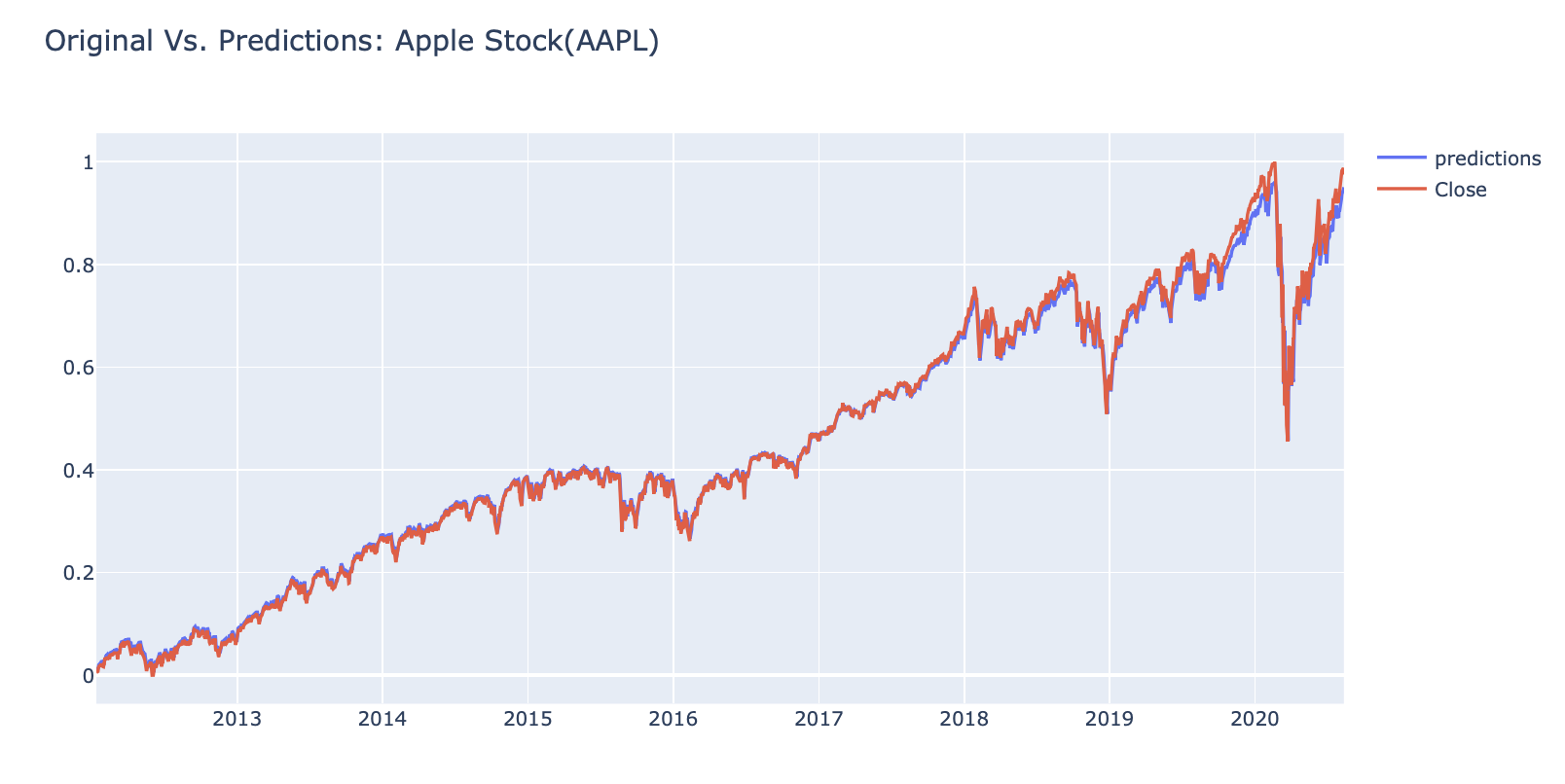# TRAIN AN LSTM TIME SERIES MODEL

#### If you want to view APPL / AMZN / ETC: Change ‘sp500 HERE!

``````# Let's test the functions and get individual stock prices and volumes for sp500
price_volume_df = individual_stock(stocks_df, stocks_vol_df, 'sp500')
``````
``````# Get the close and volume data as training data (Input)
training_data = price_volume_df.iloc[:, 1:3].values
``````
``````# Normalize the data
sc = MinMaxScaler(feature_range= (0,1))
training_set_scaled = sc.fit_transform(training_data)
``````
``````# Create the training and testing data, training data contains present day and previous day values
X = []
y = []
for i in range(1, len(price_volume_df)):
X.append(training_set_scaled[i-1:i, 0])
y.append(training_set_scaled[i, 0])
``````
``````# Convert the data into array format
X = np.array(X)
y = np.array(y)
``````
``````# Split the data
split = int(0.7 * len(X))
X_train = X[:split]
y_train = y[:split]
X_test = X[split:]
y_test = y[split:]
``````
``````# Reshape the 1D arrays to 3D arrays to feed in the model
X_train = np.reshape(X_train, (X_train.shape, X_train.shape, 1))
X_test = np.reshape(X_test, (X_test.shape, X_test.shape, 1))
``````
``````# Create the model
inputs = keras.layers.Input(shape=(X_train.shape, X_train.shape))
x = keras.layers.LSTM(150, return_sequences= True)(inputs)
x = keras.layers.Dropout(0.3)(x)
x = keras.layers.LSTM(150, return_sequences=True)(x)
x = keras.layers.Dropout(0.3)(x)
x = keras.layers.LSTM(150)(x)
outputs = keras.layers.Dense(1, activation='linear')(x)

model = keras.Model(inputs=inputs, outputs=outputs)
model.summary()
``````
``````Model: "model"
_________________________________________________________________
Layer (type)                 Output Shape              Param #
=================================================================
input_1 (InputLayer)         [(None, 1, 1)]            0
_________________________________________________________________
lstm (LSTM)                  (None, 1, 150)            91200
_________________________________________________________________
dropout (Dropout)            (None, 1, 150)            0
_________________________________________________________________
lstm_1 (LSTM)                (None, 1, 150)            180600
_________________________________________________________________
dropout_1 (Dropout)          (None, 1, 150)            0
_________________________________________________________________
lstm_2 (LSTM)                (None, 150)               180600
_________________________________________________________________
dense (Dense)                (None, 1)                 151
=================================================================
Total params: 452,551
Trainable params: 452,551
Non-trainable params: 0
_________________________________________________________________
``````
``````# Train the model
history = model.fit(X_train, y_train, epochs= 20, batch_size= 32, validation_split= 0.2)
``````
``````Epoch 1/20
38/38 [==============================] - 7s 55ms/step - loss: 0.0539 - val_loss: 0.0653
Epoch 2/20
38/38 [==============================] - 0s 8ms/step - loss: 0.0095 - val_loss: 0.0055
Epoch 3/20
38/38 [==============================] - 0s 11ms/step - loss: 0.0012 - val_loss: 5.9507e-04
Epoch 4/20
38/38 [==============================] - 0s 10ms/step - loss: 3.8284e-04 - val_loss: 2.3346e-04
Epoch 5/20
38/38 [==============================] - 0s 9ms/step - loss: 3.5239e-04 - val_loss: 8.1833e-05
Epoch 6/20
38/38 [==============================] - 0s 10ms/step - loss: 3.5036e-04 - val_loss: 6.2046e-05
Epoch 7/20
38/38 [==============================] - 0s 8ms/step - loss: 3.0313e-04 - val_loss: 4.0566e-05
Epoch 8/20
38/38 [==============================] - 0s 9ms/step - loss: 2.8564e-04 - val_loss: 6.2951e-05
Epoch 9/20
38/38 [==============================] - 0s 9ms/step - loss: 3.1342e-04 - val_loss: 5.7098e-05
Epoch 10/20
26/38 [===================>..........] - ETA: 0s - loss: 2.9808e-04
``````
``````# Make prediction
predicted = model.predict(X)
``````
``````test_predicted = []

for i in predicted:
test_predicted.append(i)
``````
``````df_predicted = price_volume_df[1:][['Date']]
``````
``````df_predicted['predictions'] = test_predicted
``````
``````close = []
for i in training_set_scaled:
close.append(i)
``````
``````df_predicted['Close'] = close[1:]
df_predicted
``````
``````# Plot the results
#interactive_plot(df_predicted, 'Original Vs Predictions: SP500')
``````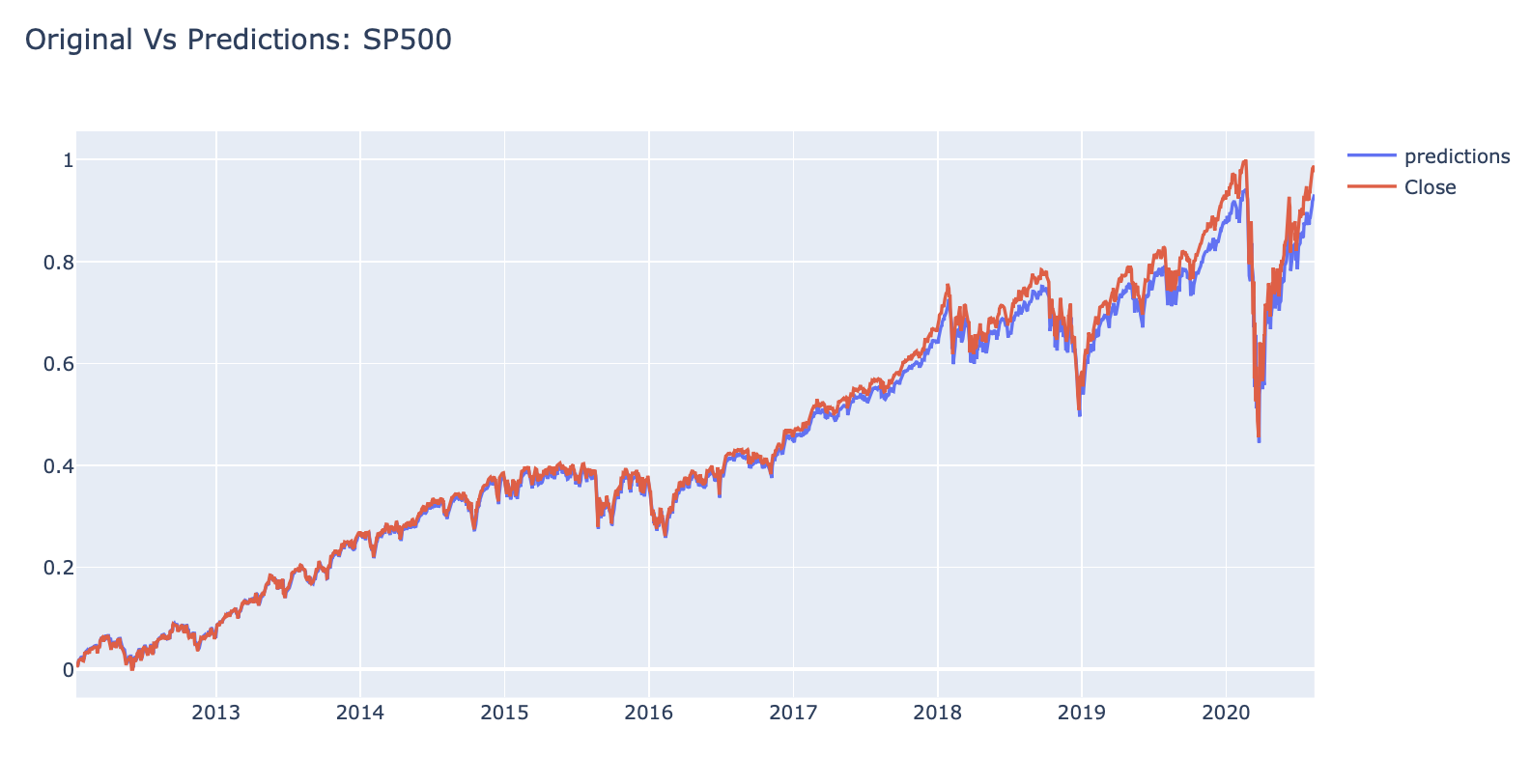##### Andrew D'Armond

Leveraging data science to achieve results